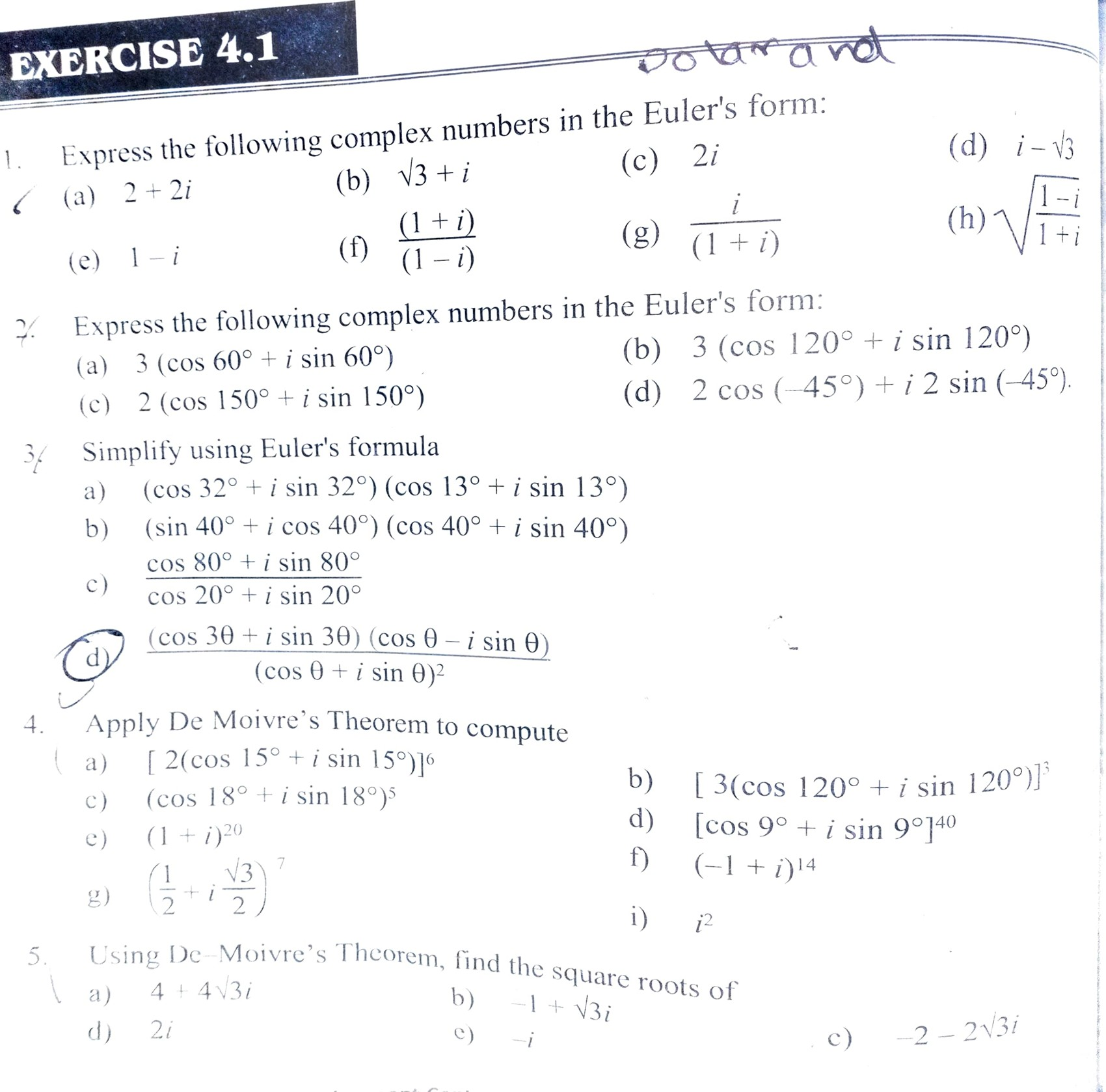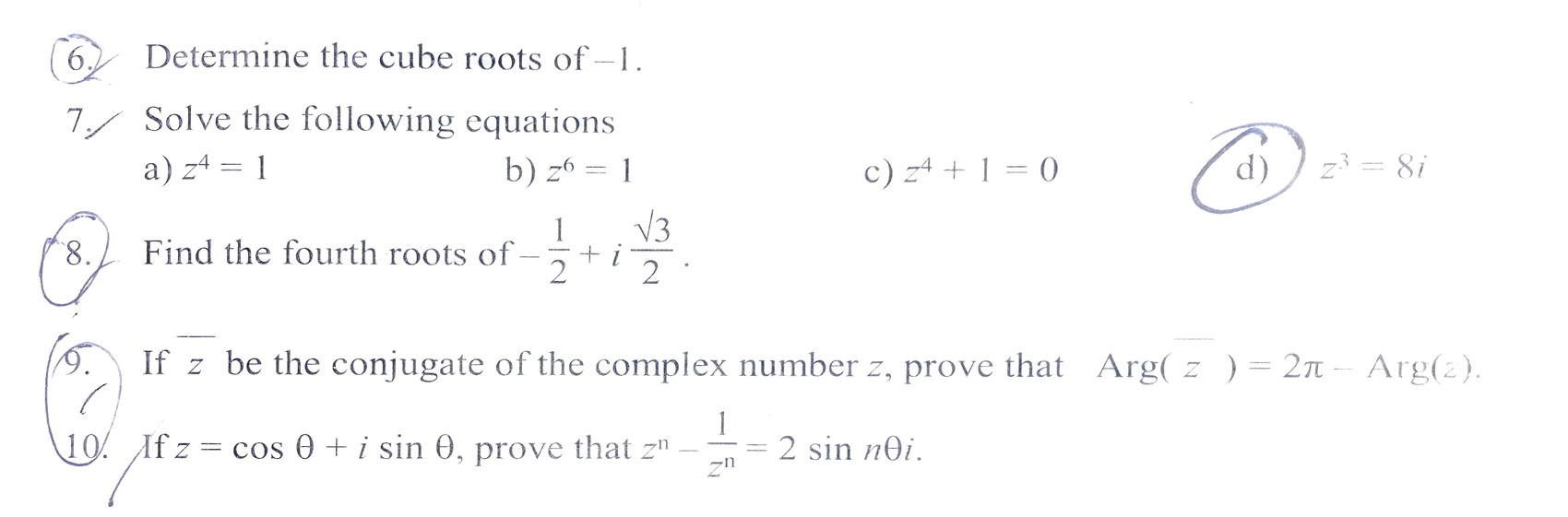# Complex Number - Exercise 4.1 : Class 12 Math

Complete Exercise 4.1 Complex Number. Exercise 4.1: Complex Number Complete Question Answer Exercise Solution note, Class 12 Mathematics.

## Chapter 4: Complex Number.

### Exercise: 4.1

Complete Exercise of Complex Number - Exercise 4.1 : Class 12 Mathematics 2080 NEB.

Exercise 4.1 is about: Polar Form of a Complex Number, Eular's Formula, Products and Quotients in Polar Form, Intergral Powers and Roots of Complex Numbers, De Moivre's Theorem, and Root of a given complex number.

#### Complex Number - Exercise 4.1 QuestionsGetting Info...